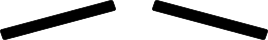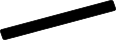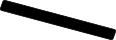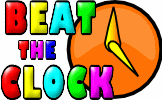# Fickle Fractions

## Compare pairs of fractions to identify the largest or smallest in order to move through the maze. Earn a trophy for finding the right door.

##### Level 1Level 2Level 3Level 4Level 5DescriptionHelpMore FractionsClick on the smallest
of these two fractions$$\frac{5}{10}$$

$$\frac{2}{5}$$

SMALLEST

$$\frac{1}{5}$$

$$\frac{4}{8}$$

LARGEST

$$\frac{1}{3}$$

$$\frac{3}{5}$$

LARGEST

$$\frac{3}{6}$$

$$\frac{2}{7}$$

SMALLEST

$$\frac{4}{9}$$

$$\frac{2}{6}$$

SMALLEST

$$\frac{5}{7}$$

$$\frac{2}{5}$$

LARGEST

$$\frac{5}{7}$$

$$\frac{1}{3}$$

SMALLEST

$$\frac{1}{2}$$

$$\frac{5}{7}$$

SMALLEST

$$\frac{5}{10}$$

$$\frac{2}{7}$$

SMALLEST

$$\frac{1}{2}$$

$$\frac{3}{5}$$

SMALLEST

$$\frac{1}{4}$$

$$\frac{4}{6}$$

LARGEST

$$\frac{1}{3}$$

$$\frac{1}{5}$$

SMALLEST

$$\frac{2}{3}$$

$$\frac{4}{8}$$

LARGEST

$$\frac{3}{4}$$

$$\frac{5}{8}$$

LARGEST

$$\frac{3}{6}$$

$$\frac{2}{7}$$

LARGEST

$$\frac{1}{4}$$

$$\frac{1}{3}$$

SMALLEST

$$\frac{4}{6}$$

$$\frac{3}{4}$$

SMALLEST

$$\frac{2}{7}$$

$$\frac{5}{10}$$

SMALLEST

$$\frac{3}{6}$$

$$\frac{5}{9}$$

SMALLEST

$$\frac{3}{6}$$

$$\frac{2}{7}$$

SMALLEST

This is Fickle Fractions level 3. You can also try:
Level 1 Level 2 Level 4 Level 5

## Transum.org

This web site contains over a thousand free mathematical activities for teachers and pupils. Click here to go to the main page which links to all of the resources available.## More Activities:

Mathematicians are not the people who find Maths easy; they are the people who enjoy how mystifying, puzzling and hard it is. Are you a mathematician?

Comment recorded on the 10 April 'Starter of the Day' page by Mike Sendrove, Salt Grammar School, UK.:

"A really useful set of resources - thanks. Is the collection available on CD? Are solutions available?"

Comment recorded on the 16 March 'Starter of the Day' page by Mrs A Milton, Ysgol Ardudwy:

"I have used your starters for 3 years now and would not have a lesson without one! Fantastic way to engage the pupils at the start of a lesson."

Each month a newsletter is published containing details of the new additions to the Transum website and a new puzzle of the month.

The newsletter is then duplicated as a podcast which is available on the major delivery networks. You can listen to the podcast while you are commuting, exercising or relaxing.

Transum breaking news is available on Twitter @Transum and if that's not enough there is also a Transum Facebook page.

#### Beat The ClockIt is a race against the clock to answer 30 mental arithmetic questions. There are nine levels to choose from to suit pupils of different abilities.

There are answers to this exercise but they are available in this space to teachers, tutors and parents who have logged in to their Transum subscription on this computer.

A Transum subscription unlocks the answers to the online exercises, quizzes and puzzles. It also provides the teacher with access to quality external links on each of the Transum Topic pages and the facility to add to the collection themselves.

Subscribers can manage class lists, lesson plans and assessment data in the Class Admin application and have access to reports of the Transum Trophies earned by class members.

Subscribe

## Go Maths

Learning and understanding Mathematics, at every level, requires learner engagement. Mathematics is not a spectator sport. Sometimes traditional teaching fails to actively involve students. One way to address the problem is through the use of interactive activities and this web site provides many of those. The Go Maths main page links to more activities designed for students in upper Secondary/High school.

## Teachers

If you found this activity useful don't forget to record it in your scheme of work or learning management system. The short URL, ready to be copied and pasted, is as follows:

Alternatively, if you use Google Classroom, all you have to do is click on the green icon below in order to add this activity to one of your classes.

It may be worth remembering that if Transum.org should go offline for whatever reason, there are mirror site at Transum.info that contains most of the resources that are available here on Transum.org.

When planning to use technology in your lesson always have a plan B!

Do you have any comments? It is always useful to receive feedback and helps make this free resource even more useful for those learning Mathematics anywhere in the world. Click here to enter your comments.For All:

Scan the QR code below to visit the online version of this activity.https://Transum.org/go/?Num=863

## Description of Levels

CloseLevel 1 - Compare simple proper fractions with a half

Level 2 - Compare proper fractions where one denominator is a multiple of the other

Level 3 - Compare proper fractions with different denominators (one denominator is not a multiple of the other)

Level 4 - Compare fractions with large numerators and denominators by simplifying the fractions first.

Level 5 - Mixed questions

Fraction Lines - Sort a number of fractions (and decimals and percentages) in a line.

More on this topic including lesson Starters, visual aids, investigations and self-marking exercises.

Answers to this exercise are available lower down this page when you are logged in to your Transum account. If you don’t yet have a Transum subscription one can be very quickly set up if you are a teacher, tutor or parent.

## Curriculum Reference

See the National Curriculum page for links to related online activities and resources.

## Help Video

You may also find a Fraction Wall useful.

Answers to this exercise are available lower down this page when you are logged in to your Transum account. If you don’t yet have a Transum subscription one can be very quickly set up if you are a teacher, tutor or parent.

Close